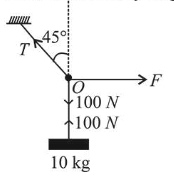# A mass of 10 kg is suspended by a rope of length 4 m,Question:

A mass of $10 \mathrm{~kg}$ is suspended by a rope of length $4 \mathrm{~m}$, from the ceiling. A force $\mathrm{F}$ is applied horizontally at the mid-point of the rope such that the top half of the rope makes an angle of $45^{\circ}$ with the vertical. Then $F$ equals: (Take $g=10 \mathrm{~ms}^{-2}$ and the rope to be massless)

1. (1) $100 \mathrm{~N}$

2. (2) $90 \mathrm{~N}$

3. (3) $70 \mathrm{~N}$

4. (4) $75 \mathrm{~N}$

Correct Option: 1

Solution:

(1) From the free body diagram$T \cos 45^{\circ}=100 \mathrm{~N}$

$T \sin 45^{\circ}=F$

On dividing (i) by (ii) we get

$\frac{T \cos 45^{\circ}}{T \sin 45^{\circ}}=\frac{100}{F}$

$\Rightarrow \quad F=100 \mathrm{~N}$